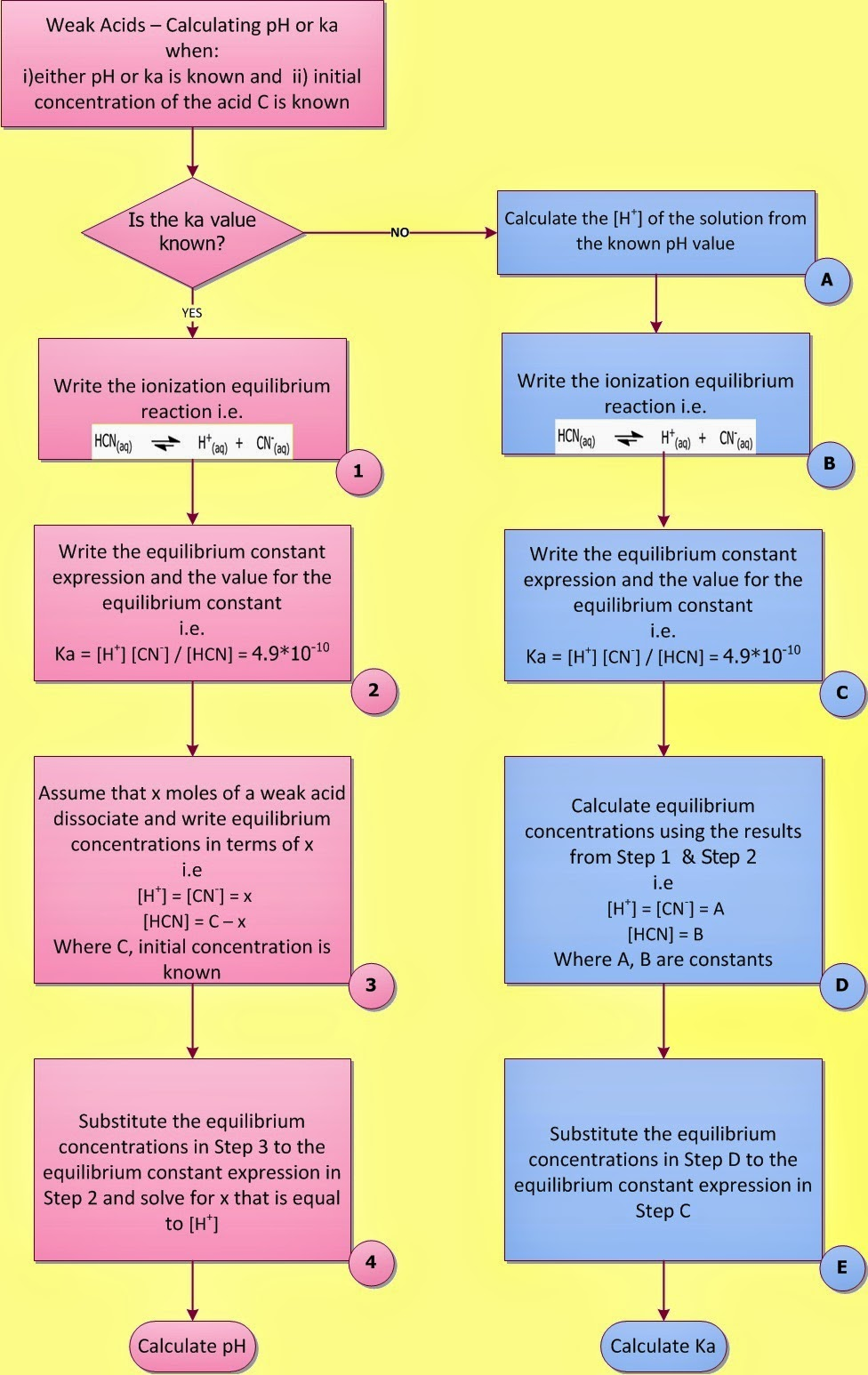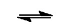Weak Acids and Bases – Calculate the pH of a weak acid (a general equation) | Chemistry Net

# Weak Acids and Bases – Calculate the pH of a weak acid (a general equation)

Weak Acids and Bases – Calculate the pH of a weak acid (a general equation)

# Weak Acids and Bases – Calculate the pH of a weak acid (a general equation)

### The weak acid (base) general equation

Most acids known are weak while there are no more than twenty strong acids. The reactions of weak acids and bases with water do not go to completion. So to calculate the pH of their solutions (pH of a weak acid or pH of a weak base ) the acid (or base) dissociation constants are used, ka and kb respectively, and the laws of chemical equilibrium.
Let us now find a general equation for the pH of a weak acid HA (or for [H+]). This is the mathematical approach.

#### Mathematical Approach:

There are two equilibria present: i) the dissociation of water and ii) the dissociation of the weak acid ka

[H+] [OH-] = kw        (1)

[H+] [A-] = ka [HA]        (2)

If the concentration of the acid in solution is C (M, moles/l), a mass balance on the anion A gives:

C = [HA] + [A-]            (3)

and a charge balance gives:

[H+] = [OH-] + [A-]             (4)

Let us assume that ka, kw  and C are known. Then there are four equations and four unknowns (shown in red in equations (1) to (4)).
A general expression for [H+] (or for the pH of a weak acid) in this case would be an expression in terms of the known ka, kw  and C. Therefore, the unknowns have to be eliminated starting from [HA] or [OH-] that are contained in the least number of equations.
Let us eliminate first [OH-]. Solving equation (1) for [OH-] and substituting it in equation (4):

[H+] [OH-] = kw   and  [OH-] = kw / [H+]   (1’)

By substituting (1’) to (4) eliminates [OH-]:

[H+] = [OH-] + [A-] = kw / [H+] + [A-]           (5)

Next let us eliminate [HA] by solving equation (3) for [HA] and substituting in equation (2):

C = [HA] + [A-]   and [HA] = C - [A-]     (3’)

Substitute (3’) in equation (2) and eliminate [HA]:

[H+] [A-] = ka [HA]  = ka (C - [A-])     (6)

Now let us eliminate [A-] by solving equation (5) for [A-] and substitute in equation (6):

[H+] = kw / [H+] + [A-]  and  [A-] = [H+] - kw / [H+]     (7)

Substituting (7) in equation (6):

[H+] [A-] = ka [HA]  = ka (C - [A-])  and

[H+] ([H+] - kw / [H+])  = ka (C -[H+] - kw / [H+]) )      (8)

Rearranging (8) we get a cubic equation in [H+]:

#### [H+]3 + ka[H+]2 – (kw + ka C) [H+] - kw ka = 0       (9)

Using the known values of C, ka, kw  equation (9) can be solved for [H+] and the pH can be calculated. The solution of the cubic equation is straightforward but tedious.
In our days, solution of equations like (9) has become easier by using on line cubic calculators.

#### Example I.1

Calculate the pH of a 0.20 M solution of HCN (ka = 4.9*10-10)

 Data [HCN]= C = 0.20 M ka = 4.9*10-10 Unknown pH = ?

The ka indicates that HCN is a weak acid. It dissociates partially in water according to:

By following the above mathematical treatment (exact solution) equation (9) becomes:

[H+]3 + (4.9*10-10)*[H+]2 – (10-14+ 4.9*10-10 *2 * 10-1)*[H+] – (10-14*4.9*10-10) =0

Solutions to equations like the above cubic equation are given by on-line cubic calculators (Fig. I.1):Fig. I.1: Three solutions to the above cubic equation are given by the Wolfram/Alpha calculator. The positive solution is the one with any physical meaning. Therefore [H+] = 9.9 * 10-6 M.

Therefore [H+] = 9.9 * 10-6 M and the pH of the solution can be calculated:

pH = -log[H+] = -log (9.9 * 10-6) = 5.0

#### Approximations on the Equation giving [H+] for weak acids

The error of equilibrium data are usually ± 5% so it is usually possible to make a number of approximations on equation (9) greatly simplifying the solution of problems like the one given in Example I.1:

Case 1

If the acid is not extremely weak or the solution very dilute, the concentration of [OH-] can be neglected in comparison to that of [H+] since [OH-] <<< [H+]. Therefore:

[H+] ([H+] - kw / [H+])  = ka (C -[H+] - kw / [H+]) )      (8)

[H+] ([H+] - kw / [H+]) = ka (C -[H+] - kw / [H+]) ) [H+] ([H+] - [OH-]) = ka (C -[H+] - [OH-] ) [H+] [H+] = ka (C -[H+])

ka = [H+]2 / C -[H+]      (8a)

Equation (8a) is a quadratic equation:

[H+]2 + ka [H+] - ka C = 0       (8a)

Case 2

If the acid is fairly concentrated (more than 10-3 M) a simplification can be achieved by making the assumption that [H+] <<< C since the acid dissociates slightly and very little [H+] is produced. Therefore equation (8a) reduces to:

ka = [H+]2 / C       [H+] ≈ (ka C)1/2       (8b)

As a general rule:
Several approximations can be made on the mass and charge balances by neglecting a concentration when it is added to another.
The approximate system of equations is solved
The results are checked for consistency with the approximations
In the final form, all approximations should introduce an error of no more than 5
If all the above show that the approximations are not appropriate the equation must be solved in its exact form.

#### Calculating ka from pH or pH from ka

In order to calculate either the ka for a weak acid (ka value) when the pH of its solution is known or the pH of a weak acid when the ka value is known the following procedure is followed (Fig. I.2):Fig. I.2: Left: Flowchart showing how to calculate the pH of a weak acid solution when ka and initial concentration C of the acid is known (1-4).   Right: Flowchart showing how to calculate the ka  of a weak acid solution when pH and initial concentration C of the acid is known (A to E)

Let’s try to solve now the problem in Example I.1 using the above step-by-step approach – let’s call it chemical approach - and compare with the mathematical approach previously used. The drawback of using the mathematical approach is that formulas have to be memorized that may lead to significant errors since they only give correct results when certain assumptions are fulfilled.

Chemical Approach:

#### Example I.1 (continued…)

Calculate the pH of a 0.20 M solution of HCN (ka = 4.9*10-10)

 Given [HCN]= C = 0.20 M ka = 4.9*10-10 Asked for pH = ?

Step 1: Write the ionization equilibrium reaction

Step 2: Write the equilibrium constant expression and the value for the equilibrium constant.

ka = [H+] [CN-] / [HCN] = 4.9*10-10

Step 3: Assume that x moles/l of HCN dissociate.  Then the concentrations of the species involved in the equilibrium are:

Step 4: Substitute the equilibrium concentrations in Step 3 to the equilibrium constant expression in Step 2 and solve for x:

ka = [H+] [CN-] / [HCN] = (x)*(x) / (0.2-x) = 4.9*10-10     (9)

x2-4.9*10-10x+9.8*10-11 = 0

This expression leads to a quadratic equation in x, which can be solved using the quadratic formula or a calculator.
However, since the value of ka is very small we can assume that x is small and so:

(0.2-x) ≈ 0.2       (10)

Then from (9) and (10):  x2 / 0.2 = 4.9*10-10    and x = [H+] = 9.9*10-6

The value for x is exactly the same with the one calculated using the mathematical approach. Therefore our assumption (0.2-x) ≈ 0.2 is correct.

Therefore, pH = -log[H+] = -log (9.9 * 10-6) = 5.0

#### Example I.2

Calculate the pH of a 0.3 M solution of acetic acid at 25 °C. (ka= 1.8 * 10-5)

STEP
RESULT
NOTES

 GIVEN [CH3COOH] = 0.3 M ka = 1.8 * 10-5 ASKED FOR pH = ?
Write down the given data and the unknown.

I

Acetic acid is a weak acid as the kα value shows and therefore it dissociates partially in water.
Write down a relation that connects given data and unknowns.

pH = -log [H+]     (1)

Write the ionization equilibrium reaction:

CH3COOHΗ+    +   CH3COO-     (2)

II

Write down the equilibrium constant expression and the equilibrium constant:

ka = [H+] [CH3COO-] / [CH3COOH] = 1.8 * 10-5   (3)

III

Assume that x mol /ℓ of acetic acid dissociate and write down  the equilibrium concentrations of the species involved in terms of x.

 CH3COOH Η+ CH3COO- Initially 0.3 Μ 0 0 Change -x M +x M +x M Final (at equilibrium) (0.3 – x) M x M x M

IV

By substituting in (3) the above equilibrium concentrations:

ka = [H+] [CH3COO-] / [CH3COOH] = x . x /(0.3-x) = 1,8 * 10-5
x2 /(0.3-x) = 1.8 *10-5  (4)

Equation (4) is a quadratic equation in x that can be solved by using a calculator or the well-known formula.
However, since acetic acid is a weak acid and dissociates slightly in water and therefore x is very small we can assume that 0,3-x ≈ 0,3

So (4) becomes:
ka =  x . x /0.3 = 1.8 * 10-5
x2 = (0.3) (1.8 * 10-5
x = 2.3 * 10-3  M
Therefore, [Η+] = x = 2,3 * 10-3 M (5)
From (1) and (5)   pH = -log [H+] = -log (2.3 * 10-3) = 2.64

For more solved weak acid and base problems please see the video and the links given below.

Relevant Posts

References

J-L. Burgot “Ionic Equilibria in Analytical Chemistry”, Springer Science & Business Media, 2012
J.N. Butler  “Ionic Equilibrium – Solubility and pH calculations”, Wiley – Interscience, 1998
D. Harvey,  “Modern Analytical Chemistry”, McGraw-Hill Companies Inc., 2000
Toratane Munegumi, World J. of Chem. Education, 1.1, 12-16 (2013)
J.N. Spencer et al., “Chemistry structure and dynamics”, 5th Edition, John Wiley & Sons, Inc., 2012
L. Cardellini, Chem. Educ. Res. Pract. Eur., 1, 151-160, (2000)

Key Terms

pH weak acid solution, weak acids, bases, how to calculate pH, calculating pH, strong and weak acids bases, pH weak acid general equation, chemistry net, dissociation of water, dissociation of weak acid, calculating ka from pH, weak acid base pH calculation